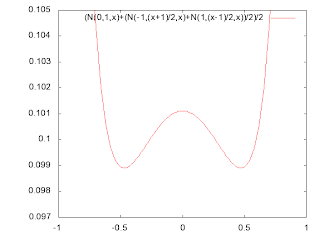## Tuesday, 14 April 2009

### Bomb Problem (part II)Assume that point A is at location -1 and point B is at 1. If one source produced both A and B and the next shot is expected to be produced by the same source, then the best fit is the normal distribution with mean 0 and dispersion 1: N(0,1,x) - gives the maximum probabilities for points A and B. On the other hand, if different sources produced A and B, the best guess would be that the third shot is normally distributed with one of the sources F(x). Now since no information present about whether one or two sources produced A and B, give the same chances to either way:

f(x)=(N(0,1,x)+F(x))/2

For F(x), give the same chances to points A and B:

F(x)=(F(-1,x)+F(1,x))/2

And the best guess for F(y,x) would be N(y,(x-y)/2,x), because the only information about the dispersion for F(y,x) is the distance between x and y, and the dispersion (x-y)/2 is the best fit. Note, that the function F(y,x) is not proper distribution for variable x, because the probability is given for fixed dispersion and it depends on x, i.e. to be normalized the dispersion has to be fixed. The overall distribution then is:

f(x)=(N(0,1,x)+(N(-1,(x+1)/2,x)+N(1,(x-1)/2,x))/2)/2

where
N(y,s,x)=1/sqrt(2Pi*s2)*Exp(-0.5(x-y)2/s2)

The graph for function f(x) is presented above. The minimum values are at points: -0.47 and +0.47. This means that the safest place is about a quarter distance from either end.

## Friday, 3 April 2009

### Kernel Density Estimation: Multikernel and Bomb problem

Given a sequence of independent identically distributed random variables X1, X2, ..., XN with common probability density function f(x), how can one estimate f(x)? (Old good KDE problem)

Let us consider the following simple question. You are on the road and you cannot go off side the road. Two bombs fall at points A and B in no particular order (for example you can see only two shell holes, but do not know which one was the first). Where is the safest place on the road if you expect the third bomb to fall? Obviously it is as far as possible from the both points. But what about the safest place between A and B? Is it the middle? Is it the place close to the point A or B?

If you think that both bombs fell from the same source and just randomly deviated from each other, then the middle point would be the most dangerous place, because it gives the highest probability that the most probable outcome is in the middle. However, if you think that those two bombs have independent sources, then the middle is the safest place because it is likely that the third bomb is sourced from either of those two. [It is the best guess because we do not have any information at this moment.] Now if you do not know if the sources are independent, somehow dependent or both are the same, then the answer is unclear.

Note that considering both sources sampled completely independent but with the bandwidth deduced from the distance between the points is the usual way for Kernel Density Estimation. The assumption that the sampled points are uncorrelated seems a bit weird, if not illogical at all. There is no relation between making distribution around points smooth and the spatial information. Doing this properly the estimator f(x) would be a sum of delta functions on points. But this is unacceptable, so the estimator is smoothed with Gaussians or some another kernels. Now if the smoothness (bandwidth) is big enough it is possible (depending on the kernel shape) that the middle is less safe than the end points. [For example see how the most dangerous place changes with the bandwidth parameter for the sum of 2 Gaussians.] In other words the probability in the middle adds equally from both sources and it can be greater than maximum probability from the source A plus probability from the source B at the point A.

If the common kernel is taken for both points, then the most probable place for the third point is the middle, which leaves end points safest - with simple shape of the kernel the middle is the most dangerous place and the further you are from the middle the safe you are; regardless whether you stand right inside the shell hole A or B, or next to it.

Personally I would hide in 1/4 of the distance between A and B from the point A or B, i.e. in the middle between the end point A or B and the middle point. What kernel does satisfy this? I do not know.